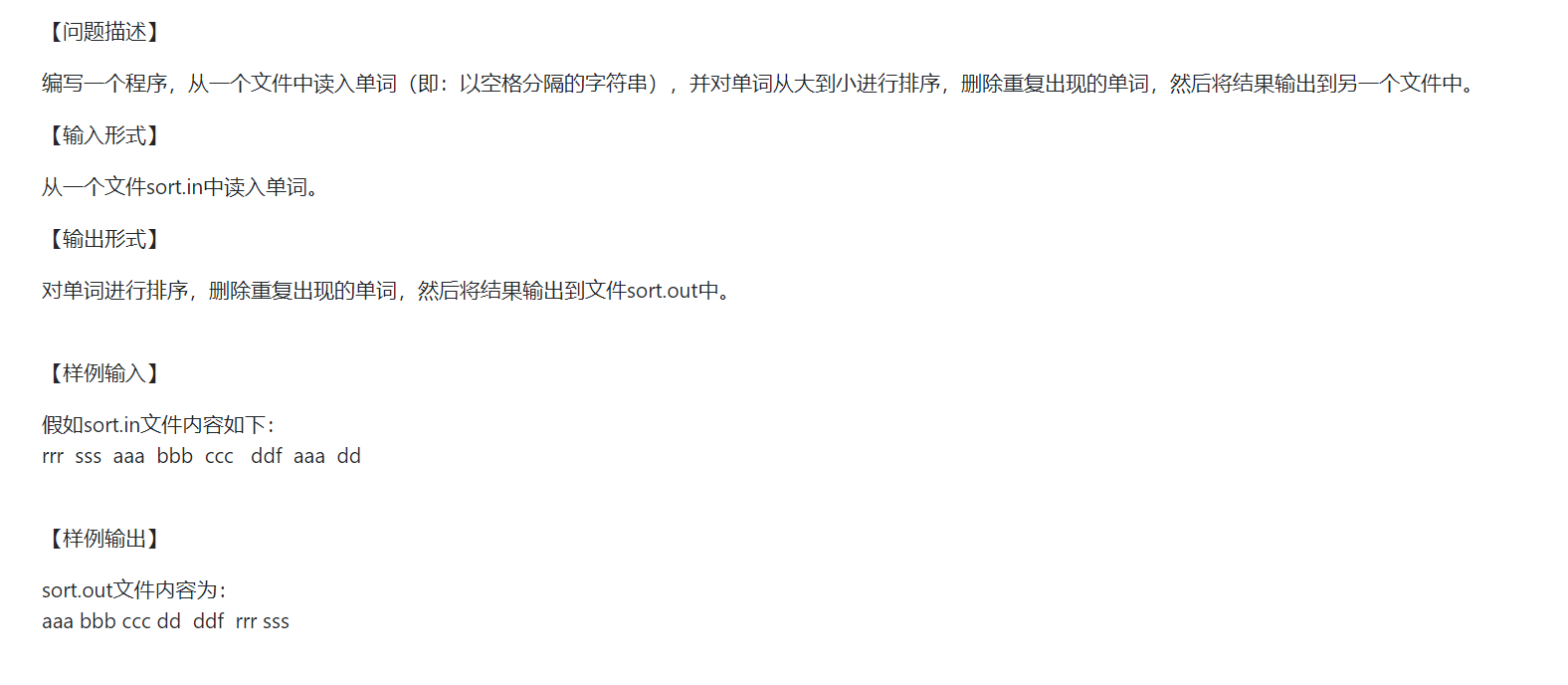2021-07-02 08:47

# c语言中文件中单词排序• 点赞
• 写回答
• 关注问题
• 收藏
• 邀请回答

#### 2条回答默认 最新

•qfl_sdu 2021-07-02 09:52
已采纳

代码如下：如有帮助，请采纳一下，谢谢。

``````#include <stdio.h>
#include <string.h>
#include <stdlib.h>

struct WordNode
{
char word;
int nmb;
struct WordNode* next;
WordNode(){next = 0;}
};

class WordCount
{
public:
~WordCount()
{
WordNode* node = 0;
{
}
}
WordNode* findNode(char *p);     //查找单词
void insertNode(WordNode* node); //插入单词
void display();   //显示
private:
};

//判断是否为空
int isEmpty(char* p)
{
while(*p)
{
if(*p > 0x20 )
return 0;
p++;
}
return 1;
}
//查找单词
WordNode* WordCount::findNode(char* p)
{
while(node)
{
if (strcmp(p,node->word) == 0)
{
return node;
}else
{
node = node->next;
}
}
return 0;
}
//插入单词
void WordCount::insertNode(struct WordNode* node)
{
//printf(" >> insert node:%s\n",node->word);
struct WordNode* P;
struct WordNode* pre;
{
return;
}
while(P)
{
if (strcmp(P->word,node->word) < 0 )  //降序排列
{
{
}else
{
pre->next = node;
node->next = P;
}
break;
}else
{
pre = P;
P = P->next;
//插入尾部
if(P==0)
{
pre->next = node;
break;
}
}
}
}
//单词的数量加1
{
node->nmb += 1;
//printf("   >> %s :%d\n",node->word,node->nmb);
}

//显示
void WordCount::display()
{
while(node)
{
printf("%s\t%d\n",node->word,node->nmb);
//cout << node->word << "\t" << node->nmb << endl;
node = node->next;
}
}
bool isctrl(char ch)
{
if (ch < 0x1F || ch == 0x7F)
{
return true;
}
return false;
}
//删除空格，并将大写转变成小写
void toLower(char tt[])
{
int i=0,j=0;
while(tt[i] != '\0')
{
if( (tt[i] != ' ') && (!isctrl(tt[i])) )
{
if(tt[i] >= 'A' && tt[i] <= 'Z')
tt[j++] = tt[i] + 32;
else
tt[j++] = tt[i];
}
i++;
}
}
//buf是存储文件的缓冲区，lSize是文件大小
{
char* buf;
FILE *pf = fopen(filename,"r");
fseek(pf,0,SEEK_END);
*lSize = ftell(pf);
// 用完后需要将内存free掉
rewind(pf);
buf = new char[*lSize+1];
buf[*lSize] = '\0';
return buf;
}

int main()
{
int size,i=0,j= 0;
char text = {0};
WordCount cc;
struct WordNode* node = 0;

if(size <= 0)
{
printf("文件打开失败，或者为空\n");
return 0;
}

while( i <= size)
{
if (i == size)
{
text[j] = '\0';
toLower(text);
if( isEmpty(text) )
{
j = 0;
i++;
continue;
}
node = 0;
node = cc.findNode(text);
if (node)
{
}else
{
node = (struct WordNode*)malloc(sizeof(WordNode));
strcpy(node->word ,text);
node->nmb = 1;
node->next = 0;
cc.insertNode(node);
}
break;
}else
{
//此处不考虑中间含有.的单词，入地名，缩写等等
if (buf[i] == ' ' || buf[i] == '\r' || buf[i] == ',' || buf[i] == '.' || buf[i] == '\n'|| buf[i] == '!' || isctrl(buf[i])|| buf[i] == ';')
{
text[j] = '\0';
toLower(text);
if(isEmpty(text))
{
j = 0;
i++;
continue;
}
node = 0;
node = cc.findNode(text);
if (node)
{
}else
{
//printf("not find,create\n");
node = (struct WordNode*)malloc(sizeof(WordNode));//new WordNode;
strcpy(node->word,text);
node->nmb = 1;
node->next = 0;
cc.insertNode(node);
}
j = 0;
}else
{
text[j++] = buf[i];
}
i++;
}
}

cc.display();

return 0;
}

``````
点赞 打赏 评论
•小P聊技术 2021-07-02 08:55
``````import java.io.*;
import java.util.*;

public class Sort
{
public static void main(String[] args) throws IOException
{
File file = new File("sort.in");
String[] strings = s.split(" +");
Set<String> stringSet = new HashSet<>(Arrays.asList(strings));
List<String> list = new ArrayList<>(stringSet);
list.sort(String::compareTo);

BufferedWriter writer = new BufferedWriter(new FileWriter("sort.out"));

for (String s1: list)
{
writer.write(s1);
if (!Objects.equals(list.get(list.size() - 1), s1))
writer.write(" ");
}
writer.close();
}
}

``````
点赞 打赏 评论